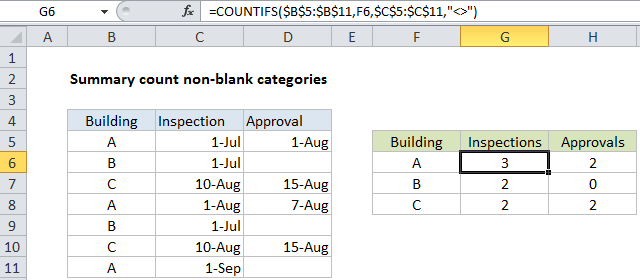## Excel Office

Excel How Tos, Tutorials, Tips & Tricks, Shortcuts

# Summary count of non-blank categories in Excel

This tutorial shows Summary count of non-blank categories in Excel using the example below;

## Formula

`=COUNTIFS(range1,criteria1,range2,"<>")`## Explanation

To build a summary count of non-blank categories, you can use the COUNTIFS function.

In the example show, the formula in G6 is:

`=COUNTIFS(\$B\$5:\$B\$11,F6,\$C\$5:\$C\$11,"<>")`

### How this formula works

This example relies on core COUNTIFS functionality.

Worked Example:   Excel Rank if formula Example

To create a summary count by building, we would use COUNTIFS like this:

`=COUNTIFS(\$B\$5:\$B\$11,F6)`

Where the criteria_range1 is B5:B11 (locked as an absolute reference so that the formula can be copied down the table and criteria1 is pulled from column F. This will return a count of all matching buildings based on column B only.

Worked Example:   Summary count with percentage breakdown in Excel

To count inspections by building, we need to extend the formula by adding an additional range/criteria pair like this:

`=COUNTIFS(\$B\$5:\$B\$11,F6,\$C\$5:\$C\$11,"<>")`

Here, criteria_range2 is C5:C11 (as an absolute reference) and criteria2 is “<>”, which means “not blank” or “not empty”. For the formula in F6, this translates to: count of entries where building is A and inspection is not blank.

Worked Example:   Break ties with helper column and COUNTIF in Excel

In a similar way, to count approvals per building, we use this formula in H6:

`=COUNTIFS(\$B\$5:\$B\$11,F6,\$D\$5:\$D\$11,"<>")`

The first range/criteria pair is the same. The second range/criteria pair simply counts non-blank entries in the approval column (D5:D11).